33 C
Jaipur
Thursday, May 6, 2021

# How do I Increment a Variable in Bash? – Linux Hint

### How much product room will fintech giants leave for startups? – TechCrunch

Incrementing or decrementing the value of a counter or an iterator is one of the most crucial tasks while using loops in any programming language. In doing so, it helps us reach the termination condition of our loop without which our loop will run infinitely. Today, our focus will be on the different methods of incrementing a variable in Bash in Linux Mint 20.

## Examples of Incrementing a Variable in Bash in Linux Mint 20:

There are different ways of incrementing a variable in Bash. We will try to expand some of the most common ones through the examples below. However, we would like to introduce you to the concepts of pre- and post-increments. In the case of the former one, the value of a variable is incremented first and then assigned to another variable, whereas, in the latter, the value of a variable is stored first and is incremented afterward. The effects of both pre-increment and post-increment will be quite evident from the first two examples. So, let’s check out the example Bash scripts.

## Example #1: Post-Incrementing a Variable:

To see the effect of post-increment, you must copy the script shown in the image below in any Bash file. You can create a Bash file in your Home directory with any name of your preference, then followed by a “.sh” extension.

In this script, we have declared a variable “x” and initialized it with the value “0”. Then we have another variable, “a”, where we assigned the post incremented value of the variable “x”. Finally, the value of the variable “a” on the terminal will be printed

To see the effect of this assignment on our output, we have to execute this script with the command shown below:

\$ bash IncrementVariable.sh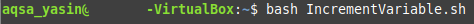Since we have post incremented the variable “x” and assigned it to the variable “a”, therefore, the value of variable “a” will still be “0”. It is so because the value of variable “x” (which was “0” initially) was first assigned to the variable “a” and then it was incremented. This output is shown in the following image: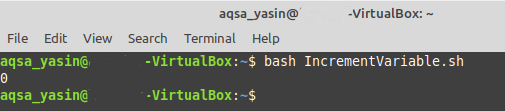## Example #2: Pre-Incrementing a Variable:

Now, for checking the effect of pre-increment, we will use the same script as shown in the example above with a slight modification, which is shown in the image below: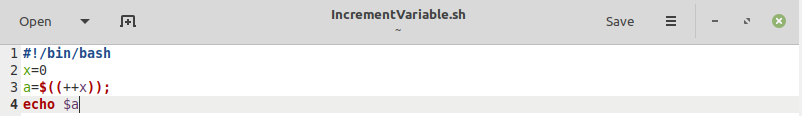In this script, instead of using post-increment, we simply used pre-increment. The remaining of the script is closely the same as example #1.

Now, when we execute this script, we will notice that the value of the variable “a” will be “1” instead of “0” because, this time, the value of the variable “x” was incremented first, and it was assigned to the variable “a”. This output is shown in the following image: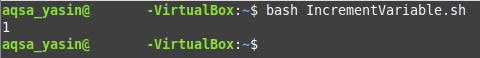## Example #3: Post-Incrementing a Variable within a “for” loop:

When you have clearly understood the concept of pre-increment and post-increment, we can use this concept within a “for” loop. The example script is shown in the image below: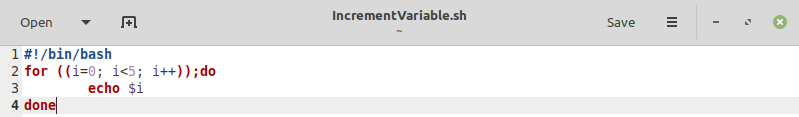In this script, there is a simple “for” loop with a counter variable or an iterator “i” whose value is being post incremented. Then we have simply printed the value of “i” for each iteration.

The output of this script is shown in the following image: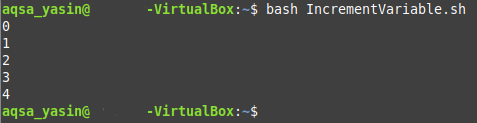## Example #4: Pre-Incrementing a Variable within a “for” loop:

For pre-incrementing a variable within a “for” loop, the example script is shown in the image below: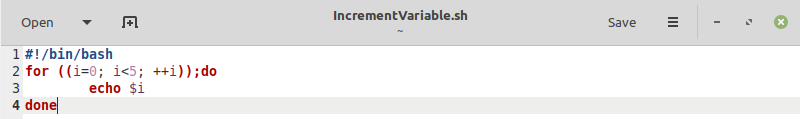This script is the same as we did in example #3. The replacement of the post-increment with the pre-increment is the sole difference between the two scripts.

The output of this script is displayed in the appended image. This output is the same as the one shown in example #3, and you might be wondering why? It is so because this time, we are not assigning the value of the variable “i” to any other variable. That is why the effects of pre-increment and post-increment have become indistinguishable in these examples.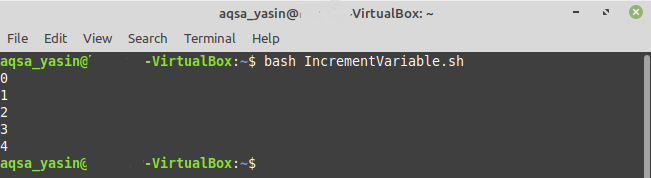## Example #5: Incrementing a Variable using “while” Loop with “+=” Notation:

The “+=” notation can also be used to increment the value of a variable and the example script demonstrated, this is shown in the image below: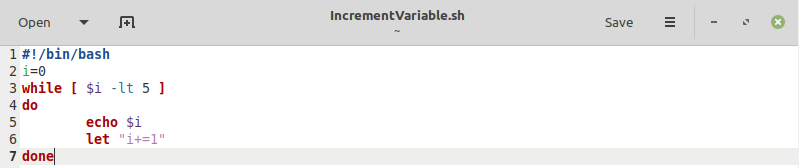In this script, we have declared a variable “i” and assigned the value “0”. Then we have a “while” loop that keeps iterating on this variable until its value is less than “5”. Within this loop, we are printing the value of this variable and then incrementing its value using the “+=” notation.

The output of this script is shown in the following image: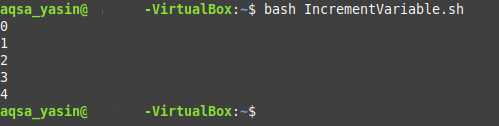## Example #6: Incrementing a Variable using “while” Loop with “+1” Notation:

The “+1” notation is also another way of incrementing the value of a variable by “1”. The example script demonstrating this is shown in the image below: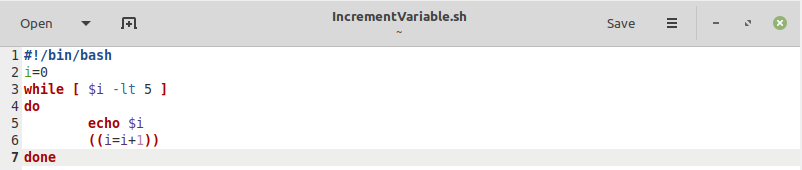This script is the same as we did in example #5. The replacement of the “+=” notation with the “+1” notation is the sole difference between the two scripts.

The output of this script is shown in the following image: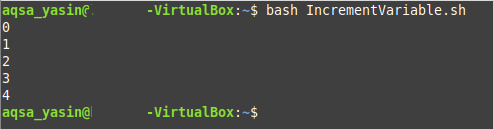## Conclusion:

In today’s tutorial, we learned six different ways of incrementing a variable in Bash. We also threw light on the concepts of pre-increment and post-increment and illustrated these concepts using suitable examples. Depending upon the functionality that you require from your program, you can either choose to pre-increment or post-increment your counter variables or iterators. Using any of the ways of incrementing variables in Bash in Linux Mint 20, you can easily increase the value of your desired variables by “1”.